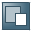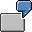Show TOC##AS Java PortsThe default AS Java ports enable you to establish communication to an AS Java instance as  part of the SAP NetWeaver cluster. The ports are generated at installation time and when new cluster elements have to be created.

For the AS Java, 20 ports are available for the ICM element and 80 are available for the server elements, since five is the maximum number of ports for a server process. Therefore, no more than 16 server cluster elements can be created on one instance.

The default AS Java ports meet the following requirements:

The port value is a number over 50000

For each cluster element the ports begin with 50000+100*instance_number, where instance_number is a two digit number from 00 to 99 specifying the number of central instance and dialog instances.You can see the instance number from the directory, where the AS Java is installed. Under /usr/sap/<SID>, there is a directory which name contains the instance name including the instance number, for example, JC40 (where 40 is the instance number).

Dispatcher port_index Values

 Index Port Name 0 HTTP port 1 HTTP SSL port 2 IIOP Initial Context port 3 IIOP SSL port 4 P4 and JMS port 5 P4 HTTP Tunneling port 6 P4 SSL port 7 IIOP port 8 Telnet port

For server cluster elements the ports are created from 50000+100*instance_number+20+n*5+port_index, where n is the number of server elements from 0 to 15 and port_index is from 0 to 4.You can see the number of the server element from the installation directory of the AS Java. Under <Drive>:\usr\sap\<SAPSID>\<Instance_Name>\j2ee\cluster, there are server<Server_Number> directories representing the server processes in the AS Java. Their number depends on the number specified at installation time.The ports for AS Java server3 with instance_number=15 are from 51535 (50000+100*15+20+3*5+0) to 51539 (50000+100*15+20+3*5+4).

Server port_index Values

 Index Port Name 0 Join port 1 Debug port

According to the formula mentioned above and the port_index values, the server ports for the AS Java are as follows:

AS Java Server Ports

 Internal Port Value Server Join Port For s0: 5NN20, for s1=5NN25, for s2=5NN30….for s15=5NN95, where s0, s1, s2,..s15 shows the number of server processes and NN is instance_number. Server Debug Port For s0: 5NN21, for s1=5NN26, for s2=5NN31….for s15=5NN96, where s0, s1, s2,..s15 shows the number of server processes and NN is instance_number.

### Example

The port for P4 on a dispatcher element with instance_number=15 is:

P4 port=50000+100*15+4=51504